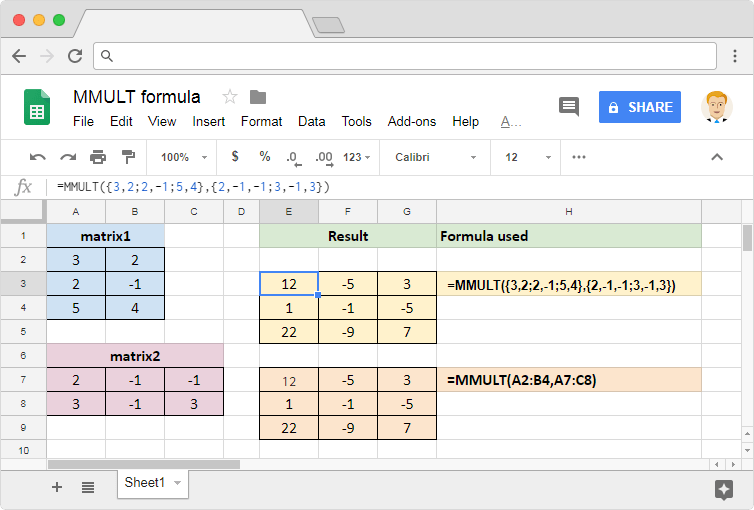# How to use the MMULT formula in Google SheetsSpreadsheets have come a long way since they were first available, thereby making our lives a lot easier. Today they provide us with a myriad of tools and formulas, which will help us with most of our mathematical and statistical calculations. Therefore, it is no surprise that we have MMULT formula in Google Sheets to help us calculate the product of two matrices.

### Syntax

MMULT(matrix1, matrix2)

• matrix1 – as the name suggests, it is the first matrix that we can represent as an array or range.
• matrix2 – is the second matrix that we can represent as an array or range.

Please note that the columns in matrix1 must be equal to the rows in matrix2. This is a rule of standard in any matrix multiplication.

### Usage: MMULT formula in Google Sheets

We will certainly need the help of a few practical examples to clearly understand the usage of this formula. So, let’s get our hands dirty in applying this in practice. Please consider the following snapshot. For the purposes of illustration, please consider the values within the range A2:B4 for matrix1, and the values in the range A7:C8 for matrix2.We’ve keyed in the first formula within the cell E3. We see that the output data flow from E3 towards the right and then further down. That is because the output of a matrix multiplication is a multidimensional array. So, it is very important that we keep the cells, where we expect the data to flow, clear of any values. Otherwise, the formula returns #REF! error.

In the first example, we used direct arrays using a pair of curly braces. We separated the values into columns and rows using commas and semi-colons respectively. In the second example, we used references to the ranges of cells that contain the values we used in the first example. Regardless of the input methods, we essentially used the same input values. That is the reason why we see the exact same output in both the examples.